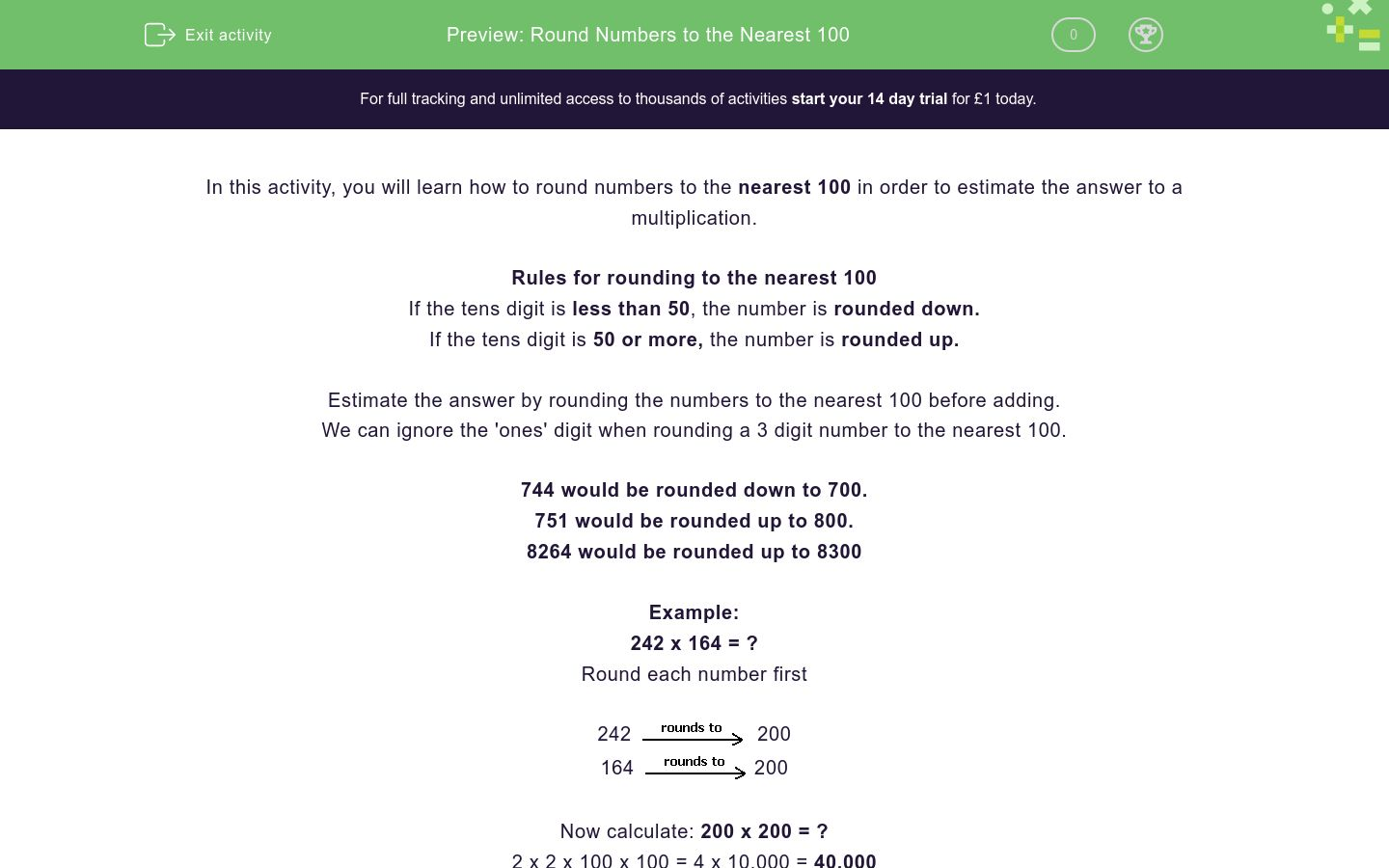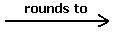# Round Numbers to the Nearest 100

In this worksheet, students will learn how to round numbers to the nearest 100 in order to estimate the answer to a calculation.Key stage:  KS 2

Curriculum topic:   Number: Addition and Subtraction

Curriculum subtopic:   Use Rounding for Answers/Accuracy

Difficulty level:### QUESTION 1 of 10

In this activity, you will learn how to round numbers to the nearest 100 in order to estimate the answer to a multiplication.

Rules for rounding to the nearest 100

If the tens digit is less than 50, the number is rounded down.

If the tens digit is 50 or more, the number is rounded up.

Estimate the answer by rounding the numbers to the nearest 100 before adding.

We can ignore the 'ones' digit when rounding a 3 digit number to the nearest 100.

744 would be rounded down to 700.

751 would be rounded up to 800.

8264 would be rounded up to 8300

Example:

242 x 164 = ?

Round each number first

242200

164200

Now calculate: 200 x 200 = ?

2 x 2 x 100 x 100 = 4 x 10,000 = 40,000

Estimate the answer by rounding the numbers to the nearest 100 before multiplying to find C.

174 x 106 =A   x   B  =  C

## Column B

A
100
B
200
C
20,000

Estimate the answer by rounding the numbers to the nearest 100 before adding to find C.

864 + 192 =A   x   B  =  C

## Column B

A
200
B
900
C
1,100

Estimate the answer by rounding the numbers to the nearest 100 before subtracting to find C.

986 - 411 =A   x   B  =  C

## Column B

A
400
B
600
C
1,000

Estimate the answer by rounding the numbers to the nearest 100 before adding.

9,648 + 1,376 = ?

11,100

11,000

12,000

10,000

Estimate the answer by rounding the numbers to the nearest 100 before multiplying.

426 x 194 = ?

Estimate the answer by rounding the numbers to the nearest 100 before subtracting.

8,784 - 6,922 = ?

1,800

1,080

1,180

1,900

John is car shopping and is trying to buy 2 cars.

He is told that the red car he likes is £3,288 and the green car he likes is £1,832.

John's total budget is £7,000.

Round the cost of each car to the nearest 100 and estimate if John has enough money.

Does John have enough money?

Yes

No

Reuben is car shopping and is trying to buy 2 cars.

He is told that the purple car he would like is £3,725

Reuben's total budget is £7,000.

Will he be able to buy 2 purple cars?

Round the cost of each car to the nearest 100 and estimate if Reuben has enough money.

Does Reuben have enough money?

No

Yes

A taxi driver is car shopping and wants to buy a fleet of new cars for his firm.

He likes a blue car that costs £1,215.

He decides to buy 7 of the blue cars.

Round the cost of each car to the nearest 100 and then estimate how much the taxi driver will spend in total.

No

Yes

A bike costs £2,312 and a yellow car costs £6,232

Will it cost more to buy four bikes or two yellow cars?

Round the cost of each vehicle to the nearest 100 and estimate the cost of buying either the bikes or the cars.

Which vehicle will cost more money?

No

Yes

• Question 1

Estimate the answer by rounding the numbers to the nearest 100 before multiplying to find C.

174 x 106 =A   x   B  =  C

## Column B

A
200
B
100
C
20,000
EDDIE SAYS
When rounded to the nearest 100, the calculation is shown as 200 x 100 = 20,000
• Question 2

Estimate the answer by rounding the numbers to the nearest 100 before adding to find C.

864 + 192 =A   x   B  =  C

## Column B

A
900
B
200
C
1,100
EDDIE SAYS
When rounded to the nearest 100, the calculation is shown as 900 + 200 = 1,100 864 you round up because 64 is greater than 50. 192 you round up because 92 is greater than 50.
• Question 3

Estimate the answer by rounding the numbers to the nearest 100 before subtracting to find C.

986 - 411 =A   x   B  =  C

## Column B

A
1,000
B
400
C
600
EDDIE SAYS
When rounded to the nearest 100, the calculation is shown as, 1000 - 400 = 600 When rounding 986 to the nearest 100, we round up as the tens digit is more than 50, this means the number is then rounded up to 1,000. Is this getting easier for you? Let's keep practising until it's second nature.
• Question 4

Estimate the answer by rounding the numbers to the nearest 100 before adding.

9,648 + 1,376 = ?

11,000
EDDIE SAYS
The rounded calculation to the nearest 100 is, 9,600 + 1,400 = 11,000 Hopefully, this is becoming less daunting for you.
• Question 5

Estimate the answer by rounding the numbers to the nearest 100 before multiplying.

426 x 194 = ?

EDDIE SAYS
How did you find rounding to the nearest 100 in order to solve this multiplication? 400 x 200 = 4 x 2 x 100 x 100= 8 x 10,000 = 80,000
• Question 6

Estimate the answer by rounding the numbers to the nearest 100 before subtracting.

8,784 - 6,922 = ?

1,800
EDDIE SAYS
The rounded calculation to the nearest 100 is 8,800 - 7,000 = 1,800
• Question 7

John is car shopping and is trying to buy 2 cars.

He is told that the red car he likes is £3,288 and the green car he likes is £1,832.

John's total budget is £7,000.

Round the cost of each car to the nearest 100 and estimate if John has enough money.

Does John have enough money?

Yes
EDDIE SAYS
John does have enough money to buy both cars. Well done if you spotted this! The rounded calculation is, 3,300 + 1,800 = 5,100. Leaving him with a remainder of £1,900 after buying both cars. Lucky John!
• Question 8

Reuben is car shopping and is trying to buy 2 cars.

He is told that the purple car he would like is £3,725

Reuben's total budget is £7,000.

Will he be able to buy 2 purple cars?

Round the cost of each car to the nearest 100 and estimate if Reuben has enough money.

Does Reuben have enough money?

No
EDDIE SAYS
Reuben does not have enough money to buy the two cars. The rounded calculation is 3,700 + 3,700 = £7,400 He has £400 too little to buy two purple cars. Poor Reuben!
• Question 9

A taxi driver is car shopping and wants to buy a fleet of new cars for his firm.

He likes a blue car that costs £1,215.

He decides to buy 7 of the blue cars.

Round the cost of each car to the nearest 100 and then estimate how much the taxi driver will spend in total.

EDDIE SAYS
£1,215 rounded to the nearest 100 = £1,200 £1,200 x 7 = £8,400 The taxi driver will spend approximately £,8400.
• Question 10

A bike costs £2,312 and a yellow car costs £6,232

Will it cost more to buy four bikes or two yellow cars?

Round the cost of each vehicle to the nearest 100 and estimate the cost of buying either the bikes or the cars.

Which vehicle will cost more money?

EDDIE SAYS
Bike cost = £2,300 x 4 = £9,200 Yellow car cost = £6,200 x 2 = £12,400 Therefore, it costs more to buy two yellow cars than four purple bikes. Great focus, that’s another activity completed!
---- OR ----

Sign up for a £1 trial so you can track and measure your child's progress on this activity.

### What is EdPlace?

We're your National Curriculum aligned online education content provider helping each child succeed in English, maths and science from year 1 to GCSE. With an EdPlace account you’ll be able to track and measure progress, helping each child achieve their best. We build confidence and attainment by personalising each child’s learning at a level that suits them.

Get started